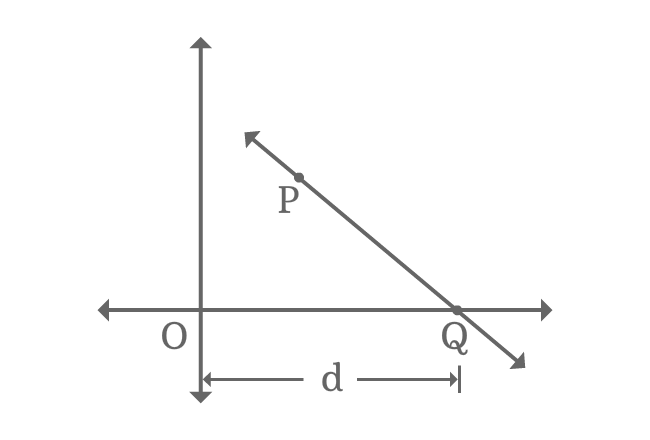# x-intercept

The distance of an intersecting point of a straight line and horizontal axis from origin is called $x$-intercept.

## Introduction

A straight line often intersects horizontal x-axis at a point. The distance from the intersecting point from origin in x-axis direction is known as $x$-intercept.

### ExampleImagine a straight line in Cartesian coordinate system. It intersects the horizontal $x$-axis at point $Q$ and $P$ is a point on the straight line.

The distance from origin to intersecting point of straight line $\overleftrightarrow{PQ}$ and $x$-axis in horizontal axis direction is $d$. The distance $d$ is called $x$-intercept.

$x$-intercept $\,=\,$ $d$

$\implies OQ$ $\,=\,$ $d$

The intersecting point $Q$ is on $x$-axis. So, its $y$-coordinate is equal to zero but its $x$-coordinate is equal to $x$-intercept. Therefore, the intersecting point $Q$ in the form of coordinates is $(d, 0)$.

Latest Math Topics
Jun 26, 2023
Jun 23, 2023

###### Math Questions

The math problems with solutions to learn how to solve a problem.

Learn solutions

Practice now

###### Math Videos

The math videos tutorials with visual graphics to learn every concept.

Watch now

###### Subscribe us

Get the latest math updates from the Math Doubts by subscribing us.